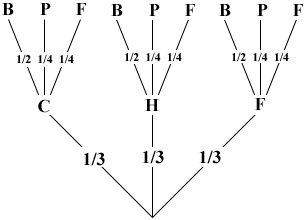SEARCH HOMEMath Central Quandaries & QueriesQuestion from Sandy, a parent: Children‚s meals are being prepared for a large gathering. There is an equal number of chicken, hamburger, and fish meals. Each meal comes with a toy: a ball, a parachute toy, or a Frisbee. There are twice as many balls as Frisbees and an equal number of parachutes and Frisbees. Use a probability tree to show the sample space and determine the probability that a meal consists of chicken or fish and comes with a ball as a toy. My daughter's been sick and missed this section I've been trying to help her but I don't know how to draw a probability either. Please helpHi Sandy,

To draw a tree diagram you think of the process happening in steps. In this case I made the first step selection of a meat and the second step selection of a toy. If these two actions are random then the probabilities of selecting chicken, hamburger or fish are each 1/3. There are twice as many balls as Frisbees and an equal number of parachutes and Frisbees so the probability of selecting a ball is 1/2 and the probability of selecting a Frisbee is 1/4 and the probability of selecting a parachute is 1/4. Here is my tree.You can now read some probabilities off the diagram by tracing a path from the root of the tree to its top. For example the probability of a child receiving a hamburger and a Frisbee is 1/3 × 1/4 = 1/12 and the probability of a child receiving fish and a ball is 1/3 × 1/2 = 1/6. Thus the probability that a child receives either a hamburger and a Frisbee or fish and a ball is 1/12 + 1/6 = 1/4.

I hope this helps,
PennyMath Central is supported by the University of Regina and The Pacific Institute for the Mathematical Sciences.